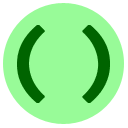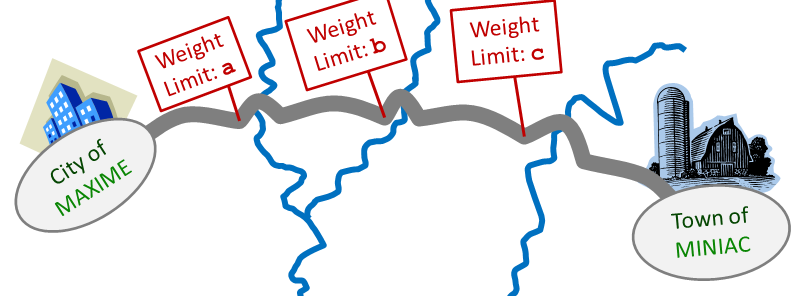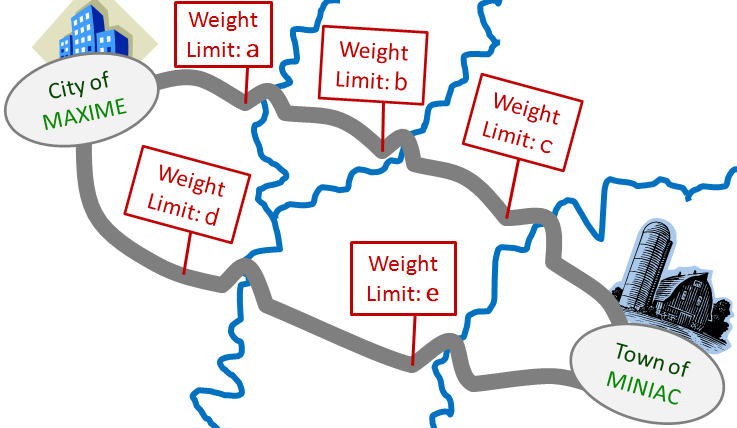# 2: Functions

We have seen one function already, `print()`, which outputs a message. To use a function you always write its name, followed by some arguments in parentheses `()`. The word argument basically means an input to the function. Then, the function does some action depending on its arguments. When there are multiple arguments to a function, you separate them with commas (`,`). For example, you can give multiple arguments to `print`; it will print all of them in order, with spaces separating them. We demonstrate in the example below.

Example
Print three numbersThe extra spaces in the sample program above had no effect on the output. Extra spaces are meaningless in most other situations too. However, be careful that extra space at the beginning of a line, called indenting, has a special meaning that can cause errors (click for an example) if used incorrectly. You will see correct indenting a few lessons later.

A function may also give back a value (like an output). For example the function `max()` (short for maximum) gives back the largest out of all of its arguments, which must be numbers.

Example
Try to predict the output of the following program, before you run it.
The name return value means the value that a function gives back. For example in `max(42, 17)` we say that "the function `max` returned the value `42`."

The `max` function has a friend which behaves similarly: the `min` function returns the minimum (smallest) of its arguments.Multiple Choice Exercise: Min and Max I
What is the output of the following program?

`x = 13y = 7a = max(x+y, x*2)b = min(x, y)print(a,b)`
Correct!

Functions can be combined to create more complicated expressions.Short Answer Exercise: Min and Max II
What is the output of the following program? Hint

`x = min(max(13, 7), 9)print(x)`
Correct!

You are not limited to using functions that are pre-defined in Python. In a few lessons you will learn how to define new functions!

## Common Errors

If you call a function with not enough arguments (inputs) or too many arguments, you get an error. For example, `max` requires at least one input:

Example
Calling `max` with too few arguments.

It's very important to carefully read the errors that you get back, when your code doesn't work. Python will usually give you helpful feedback on what went wrong. However, sometimes you need to look around a little bit to diagnose the problem — here's an example.

Example
Press Run program and look at the error that occurs.

Python says there is a syntax error, which means it can't understand what you're trying to do:

``` Traceback (most recent call last):
In line 2 of the code you submitted:
bigger = max(3, 4)
^
SyntaxError: invalid syntax```
However, the line `bigger = max(3, 4)` is actually fine. The problem actually spilled over from the previous line: we forgot to add the closing parenthesis `)` after `smaller = min(14, 99` and Python started looking at the next line for the `)`. So, check the lines before and after what Python suggests, if you are stuck debugging your programs.

## Exercise

This is a two-part exercise using the `min` and `max` functions. There are connections between the cities of Maxime and Miniac with several bridges. There is a separate limit on the amount of weight that can be transported across each bridge.For part 1, there is a single road between the two cities. The road has three bridges with weight limits `a``b``c`, as shown in the picture below:In order to drive along the route, your truck needs to drive first over the bridge with weight limit `a`, then the one with weight limit `b`, then the one with weight limit `c`. Your truck will crash if you overload any of the three weight limits. Write a program that prints out the maximum weight that can be transported along this road. Your code should assume that the variables `a``b`, and `c` already contain the bridge weight limits.Part #2: Now we will tell you the whole story. There is also a second route consisting of two bridges, the first with weight limit `d`, and the second with weight limit `e`, as illustrated below.Your truck can take either route. Write a program that prints out the maximum weight that can be transported between the two cities. Assume that the variables `a``b``c``d`, and `e` contain the bridge weight limits. Hint Hint 2

## Exercise: Code Scramble

Here is another code scramble, where you must drag-and-drop the lines to rearrange them into a correct program.Scramble Exercise: Sort of Strangeness
Arrange the code so that it prints the three numbers `x``y` and `z` sorted in increasing order, so that the smallest is printed first, then the middle one, and then the largest one.
Drag and drop with your mouse to rearrange the lines.
• print(max(x, y, z))
• print(min(x, y, z))
• print(x+y+z-min(x, y, z)-max(x, y, z))

Once you finish the exercise above, you have two choices:

• You can go directly to Lesson 3, or
• you can do some extra practice exercises about functions in Lesson 2X.